# Estimating B2B Q and Offsets

library( binfunest)
set.seed( 31394)

# Bit Error Rate Functions

The package include a number of functions that report the theoretical bit error rate (BER) of many common modulation formats, with the signal-to-noise ratio (SNR) specified as the energy in a single bit divided by the noise power in a one Hertz bandwidth ($$E_b/N_0$$). See help(“Theoretical”) for a complete list.

plot( QPSKdB, 3, 20, log="y", ylim=c( 1e-10, 0.5), panel.first = grid(),
main="Modulation Performance Curves", xlab="SNR in dB",
ylab="BER")
curve( QAMdB.8.star, 3, 20, col="blue", add=TRUE)
curve( PSQPSKdB, 3, 20, col="red", add=TRUE)
curve( QAMdB.16, 3, 20, col="green", add=TRUE)
legend( "bottomleft", legend=c( "QPSKdB", "QAMdB.8.star", "PSQPSKdB",
"QAMdB.16"),
lty=c( 1, 1, 1, 1),
col=c("black", "blue", "red", "green"))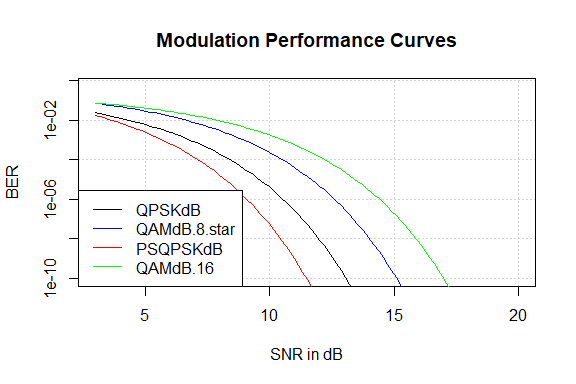# Offset and a Back-to-Back SNR

In high performance communications systems it is common for both the transmitter (Tx) and receiver (Rx) to add noise to the signal. Unless you have a perfect example of one or the other, it is impossible to measure the source: both the Tx and Rx will contribute. This has the effect of having a minimum bit error rate (BER) even when no noise is added to the signal, called the Back-to-Back (B2B) BER, and is often expressed in Decibels as the signal-to-noise ratio (SNR) which would the B2B BER in a perfect Rx.

The other common effect is to add an offset to the SNR. That is, the system may always perform as though the SNR were a few Decibels less.

We can take a BER function in Decibels and add the offset and B2B BER to it as follows. The linear SNR is $$\gamma = E_b/N_0$$, but in the B2B case the input noise $$N$$ is zero, yet there is still a finite error rate observed. This is modeled as $$\gamma = E_b/{(N_0+\beta)}$$ where $$\beta$$ is $$1/B2B$$.

$P_{B2B}(\gamma) = P\left[ O\left( \frac{1}{1/\gamma + 1/B}\right)\right]$

where $$O$$ is the linear offset (i.e., undB( offset)), $$B$$ is the linear B2B SNR, and $$P_{B2B}(\gamma)$$ is the probability of error at a linear SNR of $$\gamma$$.

## Creating BER Functions with B2B & Offset

If we have a function $$P_{dB}( s_{dB})$$ of the SNR in Decibels, we can express the B2B and offset in decibels too.

$P_e( s_{dB}, B_{dB}, O_{dB}) = BER( -dB( undB( -s_{dB}) + undB( -B_{dB}) - O_{dB})$

The B2BQ package provides a function factory to convert any such function $$P_{dB}$$ into a function with B2B and Offset in Decibels B2BConvert.

QPSKdB.B2B <- B2BConvert( QPSKdB)
O1 <- 3
B1 <- 16
s <- 0:20
plot( s, y=QPSKdB( s), typ="l", log="y", ylim=c( 1e-10, 0.5),
panel.first = grid(), main="QPSK Performance Limits", xlab="SNR in dB",
ylab="BER")
lines( s, y=QPSKdB.B2B( s, Inf, O1), col="blue", lwd=2)
lines( s, y=QPSKdB.B2B( s, B1, O1), col='red')
lines( s, y=QPSKdB.B2B( rep( B1, length( s)), Inf, O1), col='black', lty=2)
legend( "bottomleft", legend=c( "Theory", "Offset", "Offset + B2B", "B2B"),
lty=c( 1, 1, 1, 2), lwd=c(1, 2, 1, 1),
col=c( 'black', 'blue', 'red', 'black'))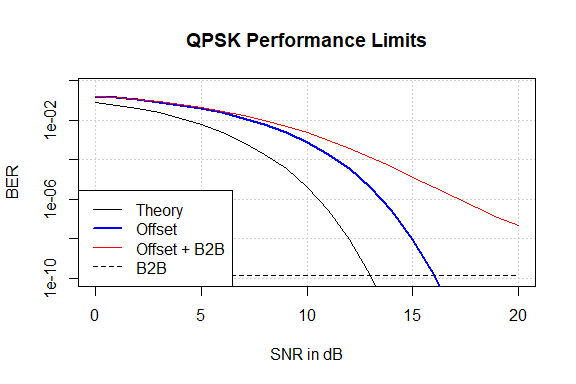Dotted line shows B2B limiting BER.

## Using nls

Let’s generate some example data and use nls to fit the curve to the data.

N <- 1000000
(r <- rbinom( length( s), N, QPSKdB.B2B( s, B1, O1)))
#>   161193 134359 107870  83728  61830  43368  28246  17266   9817   5083
#>    2195   1024    393    134     44     11      7      1      0      0
#>       0
bplot1 <- function( s, r, N, O, B, ylim=c( 1e-10, 0.5)) {
plot( s, y=QPSKdB.B2B( s, B, O), col='red', log='y', type='l', ylim=ylim,
main="QPSK Performance Limits", xlab="SNR in dB",
ylab="BER", panel.first = grid())
points( s, r/N)
lines( s, y=QPSKdB( s))
lines( s, y=QPSKdB.B2B( s, Inf, O), col="blue", lwd=2)
lines( s, y=QPSKdB.B2B( rep( B1, length( s)), Inf, O1), col='black', lty=2)
legend( "bottomleft",
legend=c( "Data", "Theory", "Offset", "Offset + B2B", "B2B"),
lty=c( NA, 1, 1, 1, 2), lwd=c(NA, 1, 2, 1, 1), pch=c( 1, NA, NA, NA, NA),
col=c( 'black', 'black', 'blue', 'red', 'black'))
}
bplot1( s, r, N, O1, B1)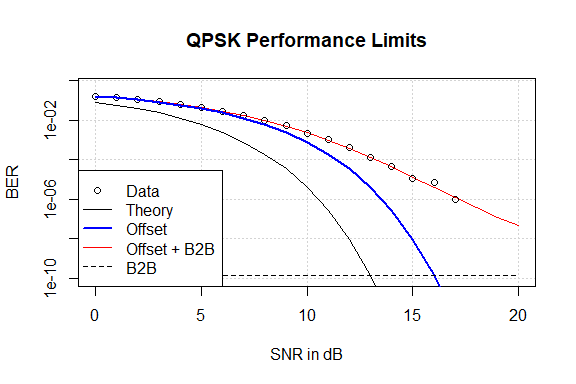Samples above 17 dB resulted in zero errors (in this example) and so are not plotted.

nls creates a non-linear least squares fit to estimate the parameters.

df1 <- data.frame( Errors=r, Trials=rep( N, length( s)), SNR=s)
nls.fit1 <- nls( Errors/Trials ~ QPSKdB.B2B( SNR, b, o), data=df1,
start=list( b=10, o=0))
summary( nls.fit1)
#>
#> Formula: Errors/Trials ~ QPSKdB.B2B(SNR, b, o)
#>
#> Parameters:
#>    Estimate Std. Error t value Pr(>|t|)
#> b 15.948539   0.052466     304   <2e-16 ***
#> o  2.992966   0.002874    1041   <2e-16 ***
#> ---
#> Signif. codes:  0 '***' 0.001 '**' 0.01 '*' 0.05 '.' 0.1 ' ' 1
#>
#> Residual standard error: 8.646e-05 on 19 degrees of freedom
#>
#> Number of iterations to convergence: 7
#> Achieved convergence tolerance: 5.708e-08
bplot1(s, r, N, O1, B1)
c <- coef( nls.fit1)
lines( s, y=QPSKdB.B2B( s, c['b'], c['o']), col='green')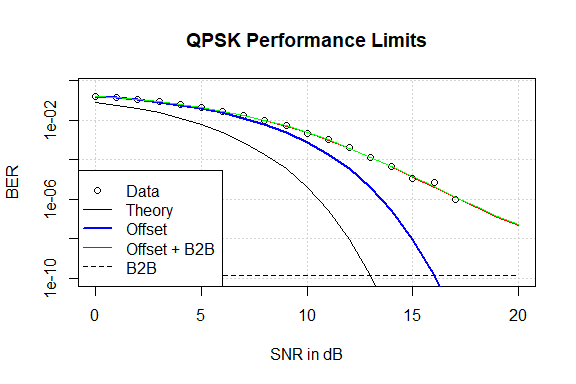Non-linear least squares fit (green line) of the line to the data.

Those are remarkably close estimates. However, we have fit the error rate to the function, not the error counts. There are zeros in the data, and this doesn’t match the function at high SNRs very well. The following will perform the fit without the zero points.

nls.fit2 <- nls( Errors/Trials ~  QPSKdB.B2B( SNR, b, o),
data=subset( df1, Errors > 0), start=list( b=10, o=0))
summary( nls.fit2)
#>
#> Formula: Errors/Trials ~ QPSKdB.B2B(SNR, b, o)
#>
#> Parameters:
#>    Estimate Std. Error t value Pr(>|t|)
#> b 15.948539   0.057174   278.9   <2e-16 ***
#> o  2.992966   0.003132   955.5   <2e-16 ***
#> ---
#> Signif. codes:  0 '***' 0.001 '**' 0.01 '*' 0.05 '.' 0.1 ' ' 1
#>
#> Residual standard error: 9.422e-05 on 16 degrees of freedom
#>
#> Number of iterations to convergence: 7
#> Achieved convergence tolerance: 1.248e-07
bplot1(s, r, N, O1, B1)
c <- coef( nls.fit2)
lines( s, y=QPSKdB.B2B( s, c['b'], c['o']), col='green')Non-linear fit (green line) excluding the zeros.

That was unsatisfying; the answer is almost identical to the version including the zeros. Plotting the data on a linear scale indicates why.

 plot( s, y=QPSKdB.B2B( s, B1, O1), col='red', type='l', panel.first = grid(),
main="QPSK Performance Limits", xlab="SNR in dB", ylab="BER")
points( s, r/N, panel.first = grid())
lines( s, y=QPSKdB( s))
lines( s, y=QPSKdB.B2B( s, Inf, O1), col="blue", lwd=2)
lines( s, y=QPSKdB.B2B( s, c['b'], c['o']), col='green')
legend( "bottomleft",
legend=c( "Data", "Theory", "3 dB Offset", "Offset + B2B", "Fit"),
lty=c( NA, 1, 1, 1, 1), lwd=c(NA, 1, 2, 1, 1), pch=c( 1, NA, NA, NA, NA),
col=c( 'black', 'black', 'blue', 'red', 'green'))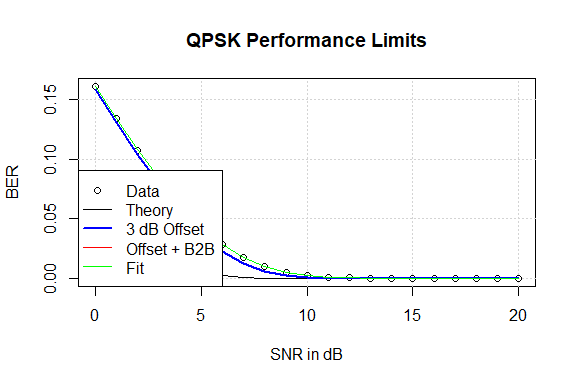Linear plot of performance showing how close to zero the estimates lie.

The error rate is so close to zero past 10 dB that a least squares error minimization is not affected by any fit errors. A better technique might be to fit the “Q” function of both sides of the equation. The Q function is Markum’s Q function and is given by

$Q(s) = \frac{1}{2} \mathrm{Erfc} \left( s / \sqrt{2}\right)$

In R, this is simply pnorm( x, lower.tail=FALSE), although the package provides the function Q_(x) for convenience. Since the Q function of zero is infinite, we have to exclude zero results again.

nls.fit3 <- nls( Q_( Errors/Trials) ~  Q_( QPSKdB.B2B( SNR, b, o)),
data=subset( df1, Errors > 0), start=list( b=10, o=0))
summary( nls.fit3)
#>
#> Formula: Q_(Errors/Trials) ~ Q_(QPSKdB.B2B(SNR, b, o))
#>
#> Parameters:
#>    Estimate Std. Error t value Pr(>|t|)
#> b 15.948434   0.057152   279.1   <2e-16 ***
#> o  2.992959   0.003142   952.4   <2e-16 ***
#> ---
#> Signif. codes:  0 '***' 0.001 '**' 0.01 '*' 0.05 '.' 0.1 ' ' 1
#>
#> Residual standard error: 3.746e-05 on 16 degrees of freedom
#>
#> Number of iterations to convergence: 7
#> Achieved convergence tolerance: 5.456e-07
bplot1(s, r, N, O1, B1)
c <- coef( nls.fit3)
lines( s, y=QPSKdB.B2B( s, c['b'], c['o']), col='green')Results of non-linear fit (green line) to Q function. Zeros are still filtered out.

# Maximum Likelihood Estimation

In order to use all of the data, and to derive statistical significance from the estimates, we must turn to maximum likelihood estimate. This will model the parameters which based on the most likely parameters based on the observed data (including the zeros).

### Log Likelihood of observations

The likelihood is the probability of that the specific observation took place. The dbinom function returns exactly this:

dbinom( r, N, QPSKdB.B2B( s, B1, O1), log=TRUE)
#>   -6.94159646 -6.75102233 -7.28795647 -6.56415934 -6.45336248 -6.29411569
#>   -6.52783185 -5.90179937 -5.88238628 -6.01136681 -8.43372668 -5.37619530
#>  -4.34938113 -3.40025874 -2.83464924 -2.31452040 -2.84437356 -1.01822454
#>  -0.37789410 -0.12626314 -0.04583595

It is easy to see that the high-SNR observations (the last ones: the SNR ranges from zero to 20; the samples are numbered one to 21) have the highest likelihood. This is because when the SNR is high, zero errors is about the only likely event, while at a lower SNR, anything the the range of $$N p \pm 2\sqrt{N p}$$ is pretty likely (where $$N$$ is the number of samples, and $$p$$ is the probability of an error; still assuming $$p << 1$$ here), so each individual result when $N p > 0$ has a small likelihood.

## Using optim

If we have data consisting of bit errors generated by testing $$N$$ bits at a variety of SNRs we can use optim to find maximum likelihood estimates of the offset and B2B.

We have to cast the parameters as a vector to work with optim. The function llsb below creates the log-likelihood of the observations. optim will naturally find minimums, so the negative of the sum is used. Sorting the log likelihood before the sum will ensure that precision isn’t lost, although such loss of precision is “unlikely.”

llsb <- function( par)
-sum( sort( dbinom( r, N, QPSKdB.B2B( s, par, par), log=TRUE),
decreasing=TRUE))
mle1 <- optim( par=c( b2b=20, off=0), llsb, hessian=TRUE)
mle1$par #> b2b off #> 15.937961 2.992359 (mle1s <- sqrt( diag( solve( mle1$hessian))))
#>         b2b         off
#> 0.060770870 0.006420547

Those are pretty close estimates. The diagonal of the inverse of the Hessian matrix is an estimate of the variance of the parameters, so their square root is the standard deviation. The expression below will show how many standard deviations from the answers those estimates are.

c( (mle1$par["b2b"] - B1)/mle1s["b2b"], (mle1$par["off"] - O1)/mle1s["off"])
#>       b2b       off
#> -1.020865 -1.190050

Below is a simple function that will take a function created by B2BConvert and estimate the two parameters. (A few extra parameters are added for possible later use.)

mlef <- function( N, s, r, f, ..., startpar=c( b2b=20, off=0),
stopifnot( length( N) == length( s) && length( s) == length( r))
ll <- function( par) {
-sum( dbinom( r, N, f( s, par, par, ...), log=TRUE))
}
res <- optim( startpar, ll, hessian=TRUE)
}

mle2 <- mlef( rep( N, length( s)), s, r, QPSKdB.B2B)
mle2$par #> b2b off #> 15.937961 2.992359 sqrt( diag( solve( mle2$hessian)))
#>         b2b         off
#> 0.060770870 0.006420547

The output is in $par, the first being the B2B SNR, and the second being the offset. Note the names come from the startpar vector. Here is a plot with the original data and the estimated line. Note the estimated line lies on top of the the “Offset + 15 dB B2B”. bplot1(s, r, N, O1, B1) lines( s, QPSKdB.B2B( s, mle2$par, mle2par), col='green', lty=4) legend( "bottomleft", legend=c( "Data", "Theory", "3 dB Offset", "Offset + 15dB B2B", "Estimated"), lty=c( NA, 1, 1, 1, 4), pch=c( 1, NA, NA, NA, NA), col=c( 'black', 'black', 'blue', 'red', 'green'))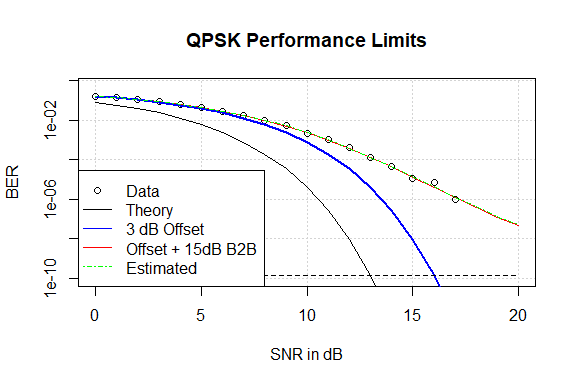Estimated as green dashed line (dashing allows the red line to peek through). ## Using mle The stats4::mle function can do a better job, and it does not need the parameters cast into a list. require( stats4) #> Loading required package: stats4 llsb2 <- function( b2b, off) -sum( dbinom( r, N, QPSKdB.B2B( s, b2b, off), log=TRUE)) mle3 <- mle( llsb2, start=c( b2b=20, off=0), nobs=length(s)) summary( mle3) #> Maximum likelihood estimation #> #> Call: #> mle(minuslogl = llsb2, start = c(b2b = 20, off = 0), nobs = length(s)) #> #> Coefficients: #> Estimate Std. Error #> b2b 15.939294 0.060817557 #> off 2.992328 0.006422466 #> #> -2 log L: 190.0448 Using stats4::mle provides other functions to work with the result as well: logLik( mle3) #> 'log Lik.' -95.02241 (df=2) vcov( mle3) #> b2b off #> b2b 0.0036987752 3.170244e-04 #> off 0.0003170244 4.124807e-05 plot(profile( mle3), absVal = FALSE)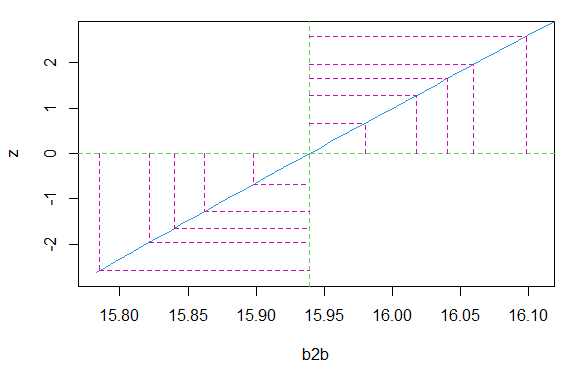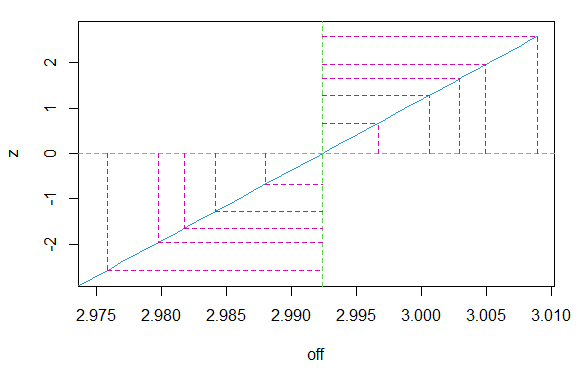confint( mle3) #> Profiling... #> 2.5 % 97.5 % #> b2b 15.821521 16.059970 #> off 2.979737 3.004913 # Using the binfunest::mleB2B The same result can be reached easier with this package’s mleB2B function, which facilitates the data being hosted in a data frame or list: df <- data.frame( Errors=r, SNR=s) (est1 <- mleB2B( data=df, Errors="Errors", N=N, f=QPSKdB.B2B, fparms=list( x="SNR"), start=c(b2b=20, offset=0))) #> #> Call: #> stats4::mle(minuslogl = function (b2b = 20, offset = 0) #> -sum(stats::dbinom(Errors, N, eval(fun), log = TRUE)), start = c(b2b = 20, #> offset = 0), method = "Nelder-Mead", nobs = 21L) #> #> Coefficients: #> b2b offset #> 15.937961 2.992359 ## Very High B2B Q It is common for many simulated systems or lower data rate systems to reach almost theoretical performance, with an offset, but no, or almost no, detectable B2B. That is, the system can be run back-to-back indefinitely and no errors. In this case, estimating a B2B might corrupt a simple offset estimate. The following has no visible B2B Q. O2 <- 3 B2 <- 24 N <- 1000000 (r2 <- rbinom( length( s), N, QPSKdB.B2B( s, B2, O2))) #>  158398 131119 104422 79027 57287 38437 23773 13422 6500 2715 #>  959 283 63 6 0 0 0 0 0 0 #>  0 mle4 <- mleB2B( Errors=r2, N=N, f=QPSKdB.B2B, fparms=list( x=s), start=c(b2b=20, offset=0)) summary(mle4) #> Maximum likelihood estimation #> #> Call: #> stats4::mle(minuslogl = function (b2b = 20, offset = 0) #> -sum(stats::dbinom(Errors, N, eval(fun), log = TRUE)), start = c(b2b = 20, #> offset = 0), method = "Nelder-Mead", nobs = 21L) #> #> Coefficients: #> Estimate Std. Error #> b2b 23.377057 0.377372172 #> offset 2.989873 0.006843009 #> #> -2 log L: 157.923 mle4coef <- coef(mle4) plot( s, r2/N, log='y', panel.first = grid(), ylim=c(1e-14, 0.5)) #> Warning in xy.coords(x, y, xlabel, ylabel, log): 7 y values <= 0 omitted from #> logarithmic plot lines( s, y=QPSKdB( s), col='black') lines( s, y=QPSKdB.B2B( s, Inf, O1), col="blue", lwd=2) lines( s, y=QPSKdB.B2B( s, B2, O2), col="red") lines( s, y=QPSKdB.B2B( s, mle4coef, mle4coef), col="green") legend( "bottomleft", legend=c( "Data", "Theory", "Offset", "Offset + B2B", "Estimated"), lty=c( NA, 1, 1, 1), lwd=c(NA, 1, 2, 1, 1), col=c( 'black', 'black', 'blue', 'red', 'green'), pch=c( 1, NA, NA, NA, NA))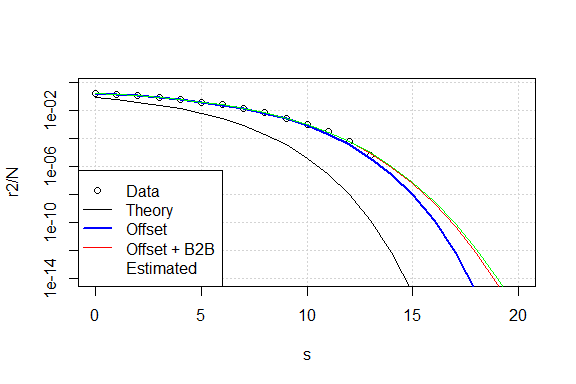24 dB B2B Q and 3 dB Offset estimates. So the plot shows the data running down the Offset line, but it is quite unconvincing that the B2B estimate is correct. Typically the B2B parameter will have a very large variance in these instances (this does not), and multiple runs will demonstrate this. Ignoring our guilty knowledge of how the data was generated, we might want to test the hypothesis that the B2B Q is really infinite (this is not an impossibility). WE can estimate the offset only using the fixed option of mle or simply setting the B2B parameter. Note that when optimizing over a single variable, a few other changes are required. (mle5 <- mleB2B( Errors=r2, N=N, f=QPSKdB.B2B, method="Brent", fparms=list( x=s, B2B=+Inf), start=c( offset=0), lower=-6, upper=10)) #> #> Call: #> stats4::mle(minuslogl = function (offset = 0) #> -sum(stats::dbinom(Errors, N, eval(fun), log = TRUE)), start = c(offset = 0), #> method = "Brent", nobs = 21L, lower = -6, upper = 10) #> #> Coefficients: #> offset #> 3.05537 AIC(mle4, mle5) #> df AIC #> mle4 2 161.9230 #> mle5 1 298.7902 The substantially lower AIC for mle4 indicates that mle5 is not a statistically better estimate. Again ignoring our guilty knowledge that the B2B Q is really 24 dB, we might now generate some more data. A 100 Gbps system that we are willing to test for an hour will generate 3.6^{14} bits, and thus could be used to test a system with a B2B Q of no more than about 24 dB (resulting in a BER of 3.6936434^{-15}, and a an expected value of 1.3297116 errors). ### Adding Observations The follow will simulate an additional run at 19 dB, and add that data to the current observations. We need to make a vector of the number of trials now as they are not all the same. N2 <- 100e9*3600 Nvec <- c( rep(N, length(s)), N2) s2 <- c(s, 19) (r4 <- c( r2, rbinom( 1, N2, QPSKdB.B2B( 19, B2, O2)))) #>  158398 131119 104422 79027 57287 38437 23773 13422 6500 2715 #>  959 283 63 6 0 0 0 0 0 0 #>  0 1 mle6 <- mleB2B( Errors=r4, N=Nvec, f=QPSKdB.B2B, fparms=list( x=s2), start=c(b2b=20, offset=0)) summary( mle6) #> Maximum likelihood estimation #> #> Call: #> stats4::mle(minuslogl = function (b2b = 20, offset = 0) #> -sum(stats::dbinom(Errors, N, eval(fun), log = TRUE)), start = c(b2b = 20, #> offset = 0), method = "Nelder-Mead", nobs = 22L) #> #> Coefficients: #> Estimate Std. Error #> b2b 23.693543 0.287762581 #> offset 2.995079 0.005659352 #> #> -2 log L: 161.4442 mle6coef <- coef(mle6) plot( s2, r4/Nvec, log='y', panel.first = grid(), ylim=c(1e-14, 0.5)) #> Warning in xy.coords(x, y, xlabel, ylabel, log): 7 y values <= 0 omitted from #> logarithmic plot lines( s2, y=QPSKdB( s2), col='black') lines( s2, y=QPSKdB.B2B( s2, Inf, O1), col="blue", lwd=2) lines( s2, y=QPSKdB.B2B( s2, B2, O2), col="red") lines( s2, y=QPSKdB.B2B( s2, mle6coef, mle6coef), col="green") legend( "bottomleft", legend=c( "Data", "Theory", "Offset", "Offset + B2B", "Estimated"), lty=c( NA, 1, 1, 1), lwd=c(NA, 1, 2, 1, 1), col=c( 'black', 'black', 'blue', 'red', 'green'), pch=c( 1, NA, NA, NA, NA))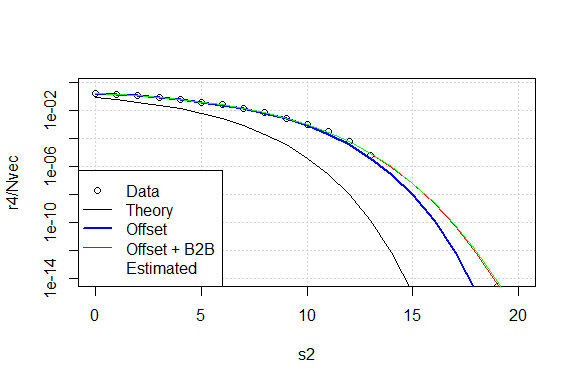24 dB B2B Q and 3 dB Offset estimates with additional data point. Note that the B2B estimate increased a bit, and the std error decreased a bit. The example below will show the same process with an infinite B2B, showing that when it truly is infinite, the AIC of an infinite model is lower. (r3 <- rbinom( length( s), N, QPSKdB( s - O2))) #>  158261 130294 103652 78825 56515 37349 22783 12445 5987 2402 #>  775 200 39 4 1 0 0 0 0 0 #>  0 mle7 <- mleB2B( N=N, Errors=r3, f=QPSKdB.B2B, fparms=list( x=s), start=c(b2b=20, offset=0)) summary(mle7) #> Maximum likelihood estimation #> #> Call: #> stats4::mle(minuslogl = function (b2b = 20, offset = 0) #> -sum(stats::dbinom(Errors, N, eval(fun), log = TRUE)), start = c(b2b = 20, #> offset = 0), method = "Nelder-Mead", nobs = 21L) #> #> Coefficients: #> Estimate Std. Error #> b2b 34.518721 5.077669338 #> offset 2.993674 0.006994309 #> #> -2 log L: 154.0507 mle8 <- mleB2B( N=N, Errors=r3, f=QPSKdB.B2B, fparms=list( x=s, B2B=+Inf), start=list(offset=0), method="Brent", lower=-6, upper=10) summary(mle8) #> Maximum likelihood estimation #> #> Call: #> stats4::mle(minuslogl = function (offset = 0) #> -sum(stats::dbinom(Errors, N, eval(fun), log = TRUE)), start = list( #> offset = 0), method = "Brent", nobs = 21L, lower = -6, upper = 10) #> #> Coefficients: #> Estimate Std. Error #> [1,] 2.998738 0.003938352 #> #> -2 log L: 154.8444 AIC(mle7,mle8) #> df AIC #> mle7 2 158.0507 #> mle8 1 156.8444 exp( (AIC( mle7) - AIC( mle6)) / 2) #>  0.02480458 # Generic Simplified Log Likelihood While the above function worked, if you don’t have substantial samples of the tail, the offset and B2B can get confused. Also, the form requires an infinite estimate for B2B if the system is performing more-or-less theoretically. We need a method that is numerically better behaved in more conditions. We can cast the BER in terms of the $$Q$$ function: $$Q( s) = (1/2)\mathrm{Erfc}(s/\sqrt{2})$$ where $$\mathrm{Erfc( x)}$$ is the complementary error function 1. Using this we can create a generic BER function as: $BER( \gamma, a, b) = Q\left( \sqrt{ \frac{a \gamma} {1 + b \gamma}} \right)$ where $$a$$ and $$b$$ are the linear versions of the offset and B2B and $$\gamma$$ is the linear SNR. We also note that R does not have the $$\mathrm{Erfc}$$, however, the following is true: Erfc( x) = 2 * pnorm( x * sqrt( 2), lower=FALSE), and the package includes the function Q_(x). With this formulation, we have the following conversions: \begin{aligned} Off_{dB} &= -dB( a) \\ B2B_{dB} &= -dB( b) \\ s_{dB} &= dB( \gamma) \end{aligned} work with the Q_ function. In order to allow the parameters to be negative without getting a negative square root, the parameters are squared in the objective function. Since our data was created with $$b = 0$$ (i.e., the B2B Q is infinite) we want the gradient search to allow negative $$a$$ and $$b$$ without failing. $BER_2( \gamma, \alpha, \beta) = Q\left( \sqrt{ \frac{\alpha^2 \gamma} {1 + \beta^2 \gamma}} \right)$ The mleB2B function will estimate $$\alpha$$ and $$\beta$$, but we will want $$a = \alpha^2$$ and $$b = \beta^2$$. Q_ab <- function( s, a, b) Q_( sqrt( a^2 * s/(1 + b^2 * s))) mle8 <- mleB2B( N=N, Errors=r2, f=Q_ab, start=c( a=1, b=0), fparms=list(s=undB(s))) summary( mle8) #> Maximum likelihood estimation #> #> Call: #> stats4::mle(minuslogl = function (a = 1, b = 0) #> -sum(stats::dbinom(Errors, N, eval(fun), log = TRUE)), start = c(a = 1, #> b = 0), method = "Nelder-Mead", nobs = 21L) #> #> Coefficients: #> Estimate Std. Error #> a 1.00232165 0.0007846674 #> b 0.06789538 0.0029126650 #> #> -2 log L: 157.906 The value of b with a comparatively large standard error is an indication that it really ought to be zero. Note that as its value is equivalent to 23.363195 dB B2BQ, . mle8coef <- coef( mle8) plot( s, y=r2/N, log='y', type='p', panel.first = grid()) #> Warning in xy.coords(x, y, xlabel, ylabel, log): 7 y values <= 0 omitted from #> logarithmic plot lines( s, QPSKdB( s)) lines( s, Q_(sqrt( undB( s)/(1 + undB( -80) * s))), col='red') lines( s, y=Q_ab( undB( s), mle8coef, mle8coef), col="green") legend( "bottomleft", legend=c( "Data", "Theory", "0 dB Offset + 80 dB B2B", "Estimated"), lty=c( NA, 1, 1, 1), col=c( 'black', 'black', 'red', 'green'), pch=c( 1, NA, NA, NA))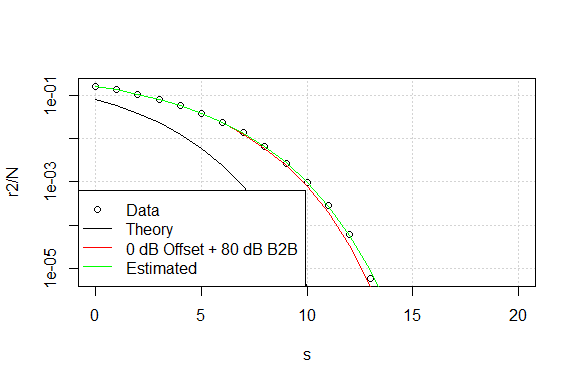That generated very nice estimates. Note that the standard deviation of $$b^2$$ is about half of $$b^2$$, so it is reasonable to let $$b = 0$$. Now let’s try it with a detectable B2B. O4 <- 3 B4 <- 15 (r4 <- rbinom( length( s), N, QPSKdB.B2B( s, B4, O4))) #>  162391 135526 109590 85323 63629 44885 29891 18587 10866 5883 #>  2964 1369 559 234 72 26 8 4 1 1 #>  1 mle9 <- mleB2B( N=N, Errors=r4, f=Q_ab, start=c( a=1, b=0), fparms=list(s=undB(s))) summary( mle9) #> Maximum likelihood estimation #> #> Call: #> stats4::mle(minuslogl = function (a = 1, b = 0) #> -sum(stats::dbinom(Errors, N, eval(fun), log = TRUE)), start = c(a = 1, #> b = 0), method = "Nelder-Mead", nobs = 21L) #> #> Coefficients: #> Estimate Std. Error #> a 0.9998006 0.0007257007 #> b 0.1767547 0.0009707055 #> #> -2 log L: 196.5237 mle9coef <- coef( mle9) (mle9sd <- sqrt( diag( vcov( mle9)))) #> a b #> 0.0007257007 0.0009707055 plot( s, r4/N, log='y',panel.first = grid()) lines( s, QPSKdB( s)) lines( s, y=QPSKdB.B2B( s, Inf, O4), col="blue") lines( s, y=QPSKdB.B2B( s, B4, O4), col="red") lines( s, y=Q_ab( undB( s), mle9coef, mle9coef), col="green", lty=5) legend( "bottomleft", legend=c( "Data", "Theory", "Theory + 3 dB", "3 dB Offset + 20 dB B2B", "Estimated"), lty=c( NA, 1, 1, 1, 5), col=c( 'black', 'black', 'blue', 'red', 'green'), pch=c( 1, NA, NA, NA, NA))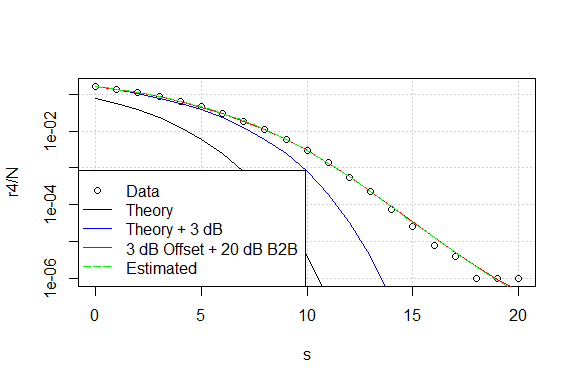We can get the coefficients from the following expression: -2*dB(mle9coef) #> a b #> 0.001732147 15.052580632 # Real Data We have some simulations of various constellations and their bit maps used with perfect detectors (i.e., the constellation points were generated, noise was added, and then the points were detected; there was no actual modulation/demodulation). The BERDFc data included with the package has a count of symbols and bits per symbol, so we need to make this data vector. Q8Sbits <- BERDFc[BERDFcName=="8QAM Star",] # Select one constellation
Q8Sbits$Nbits <- Q8Sbits$Bps * Q8Sbits\$N # Add  a Nbits column
QAMdB.8.star.B2B <- B2BConvert( QAMdB.8.star)
mle10 <- mleB2B( data=Q8Sbits, N="Nbits", Errors="BER",
f=QAMdB.8.star.B2B , start=c( B2B=30, offset=0),
fparms=list( x="SNR"))
summary( mle10)
#> Maximum likelihood estimation
#>
#> Call:
#> stats4::mle(minuslogl = function (B2B = 30, offset = 0)
#> -sum(stats::dbinom(Errors, N, eval(fun), log = TRUE)), start = c(B2B = 30,
#> offset = 0), method = "Nelder-Mead", nobs = 12L)
#>
#> Coefficients:
#>         Estimate  Std. Error
#> B2B    20.045714 0.128527020
#> offset -0.240504 0.004910512
#>
#> -2 log L: 891.1396

This looks like the B2B may be finite, and this can be verified by such a fit.

mle11 <- mleB2B( data=Q8Sbits, N="Nbits", Errors="BER",
f=QAMdB.8.star.B2B , method="Brent",
fparms=list( x="SNR", B2B=+Inf), start=c( offset=0),
lower=-6, upper=10)
summary( mle11)
#> Maximum likelihood estimation
#>
#> Call:
#> stats4::mle(minuslogl = function (offset = 0)
#> -sum(stats::dbinom(Errors, N, eval(fun), log = TRUE)), start = c(offset = 0),
#>     method = "Brent", nobs = 12L, lower = -6, upper = 10)
#>
#> Coefficients:
#>        Estimate  Std. Error
#> [1,] -0.0892018 0.002145889
#>
#> -2 log L: 2078.837
AIC( mle10, mle11)
#>       df       AIC
#> mle10  2  895.1396
#> mle11  1 2080.8373

That result indicates that the B2B is actually needed. We can see what is happening here by looking at the data.

Q8Sbits
#>         Name SNR Bps NoisePower        N    SER    BER    Nbits
#> 25 8QAM Star   3   3 0.79032060  1600000 230974 306269  4800000
#> 26 8QAM Star   4   3 0.62789043  1600000 158180 210328  4800000
#> 27 8QAM Star   5   3 0.49863525  1600000  98785 131498  4800000
#> 28 8QAM Star   6   3 0.39581559  1600000  54622  72968  4800000
#> 29 8QAM Star   7   3 0.31499330  1600000  26713  35513  4800000
#> 30 8QAM Star   8   3 0.25009840  1600000  10919  14550  4800000
#> 31 8QAM Star   9   3 0.19861226  1600000   3445   4604  4800000
#> 32 8QAM Star  10   3 0.15778619  1600000    896   1181  4800000
#> 33 8QAM Star  11   3 0.12526456  1600000    137    177  4800000
#> 34 8QAM Star  12   3 0.09954161  1600000     17     23  4800000
#> 35 8QAM Star  13   3 0.07893708  1600000      2      2  4800000
#> 36 8QAM Star  14   3 0.06276492 17600000      1      2 52800000`

The 14 dB SNR observation has only one symbol error, but reports two bit errors. This is because the 8QAM Star constellation has a few adjacent symbols that have two bit changes, and that one error doubles the estimated error rate. Therefore, this estimator indicates that the BER is not binomial distributed.

1. see Abramowitz and Stegun 29.2.29↩︎